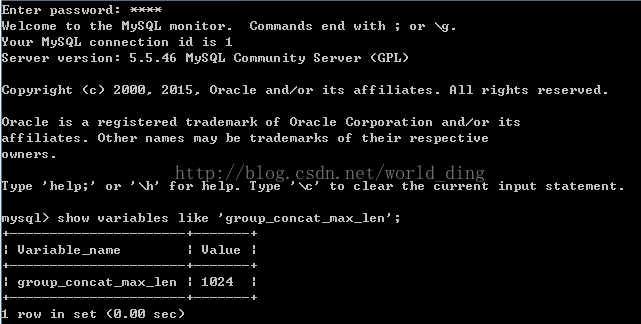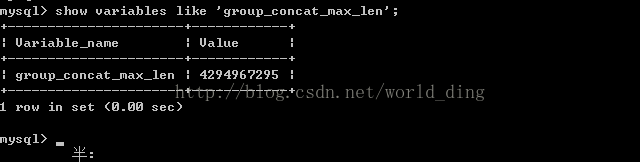# SQL query time group_ Was cut by group_ CONCAT()

When querying MySQL database, use group_ “Row XXX was cut by group_ Concat () “, check it out because of group_ There is a limit on the maximum length of concat. If it exceeds the maximum length, it will be truncated,

Let’s check the global environment variables of MySQL

``````mysql> SELECT @@global.group_concat_max_len;
+-------------------------------+
| @@global.group_concat_max_len |
+-------------------------------+
|                          1024 |
+-------------------------------+

``````

Check the maximum length of the query field in the production environment

``````mysql> select max(length(extra)) from credit.apply;
+--------------------+
| max(length(extra)) |
+--------------------+
|               9599 |
+--------------------+``````

Check the maximum number of aggregations again

``````mysql> select max(c1) from (select custid,count(1) as c1 from  credit.apply group by custid )t;
+---------+
| max(c1) |
+---------+
|      58 |
+---------+
``````

So it is estimated that the maximum possible length is

``9599 *58+67=556809``

You can change the group_ concat_ max_ Len variable solves the problem

``SET group_concat_max_len=556809;``

MySQL settings group_ concat_ max_ len

GROUP_ The concat function is used to connect multiple strings into a string. When splicing into a string, there will be the problem of splicing length. The default splicing length of MySQL is 1024 bytes. Because 1024 bytes will not be enough, sometimes it needs to be modified according to the situation. The method is as follows.

1. View the current MySQL group_ concat_ max_ len

Enter the MySQL state and enter: Show variables like ‘group_ concat_ max_ len’;

If it has not been modified, you will get the following result2. Modify MySQL group_ concat_ max_ len

a) , if it is not convenient to restart mysql, you can set it in the MySQL state through the command, such as:

`` ``
SET GLOBAL group_ concat_ max_ len = 102400;   SET SESSION group_ concat_ max_ len = 102400;

Check through mode 1.

Note: in this way, the configuration file will be read and reset after the restart of MySQL, which will lead to invalid settings, so it is recommended to modify the configuration file

b) , modify configuration file: my.ini

Add a new configuration under [mysqld]: group_ concat_ max_ len = 102400

Restart and view through mode 1.

In particular, sometimes we don’t know how many bytes we need to meet the requirements. In this case, we can consider not setting the maximum number of bytes (that is, using the maximum number of bytes), that is, setting group in the configuration file_ concat_ max_ len=-1

In this case, you can view the results in mode 1 as follows:Note: 4294967295 = 2 ^ 32 – 1